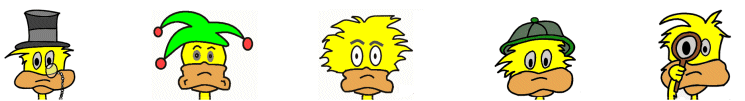Games >> Sports Games

# Math Games

## Math Genius

The object of the game is to get as many correct math answers as possible within the time limit of the game.

Instructions

A math equation will appear on the screen. The operator of the equation will be blank (or a black square). Your job is to select the operator that makes the equation correct.

Select the correct operator from the following:

"+" (plus)
"-" (minus)
"x" (times)
"÷" (divide)

You have one minute to answer as many math questions as possible. The more correct answers the better your score.

Tip: Select the Home button at the end of the game to try again.

Tip: The number of correct answers, missed answers, and accuracy are shown at the end of the game.

Tip: This game should help to improve math thought processes as well as speed of solving simple problems.

This game should work on all platforms including safari and mobile (we hope, but make no guarantees).

Back to GamesFollow us onor